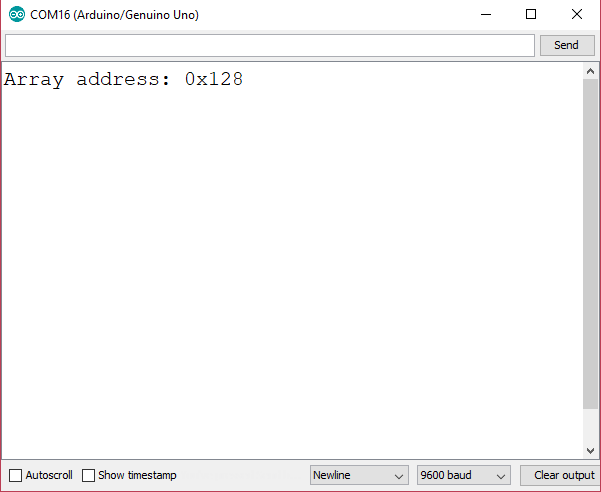# Arduino Programming: Pointers

Pointers can be confusing to beginner Arduino programmers. This tutorial aims to help you understand the concept of pointers by looking at software and hardware points of view.

### Microcontroller Memory

Pointers, as their name suggests, points to a microprocessor/microcontroller's memory. Consider the Arduino UNO board whose main microcontroller is the ATMega328p.

The ATMega328p’s memory is as follows:

• Flash Memory 32,000 bytes
• Static Random Access Memory (SRAM) 2000 bytes
• Electrically Erasable Programmable Read-only Memory (EEPROM) 1000 bytes

The flash memory stores the program or sketch. Out of its 32,000 bytes, 500 bytes are for the bootloader. The flash memory and the EEPROM are non-volatile storage areas, meaning, their data remain when the device is off.

Meanwhile, the SRAM is volatile memory and is accessed when the program is running. The SRAM contains static and global variables, dynamically allocated data items (known as heap) and local variables (known as stack).Now, what happens when we declare a variable in Arduino? Depending on the type,  a variable takes a portion of the SRAM. Their location is known through their address.

### Declaring a Pointer

Simply put, a pointer is a variable that points the address in memory of another variable. A pointer initializes like this:

`int *p;`

The above statement is useless unless we specify where it points to. Let’s say we want to point to the address of an integer variable:

`int i;`

To point to the address of i and put that address to p, we do:

`p = &i;`

### SRAM Location of a Variable

What is the location of i in SRAM if ever i is the first variable? The sketch below prints the address of i in serial monitor:

```int *p;
int i;
void setup() {
p = &i;
Serial.begin(9600);
Serial.println((uint16_t)p,HEX);
}
void loop() { }

```

[box type="info" align="aligncenter" class="" width="500px"]

`Serial.println((uint16_t)p,HEX);`

This is casting p to an unsigned 16-bit integer because the Serial.println() function can’t print pointer values directly.[/box]

Run the sketch and this shows in the serial monitor: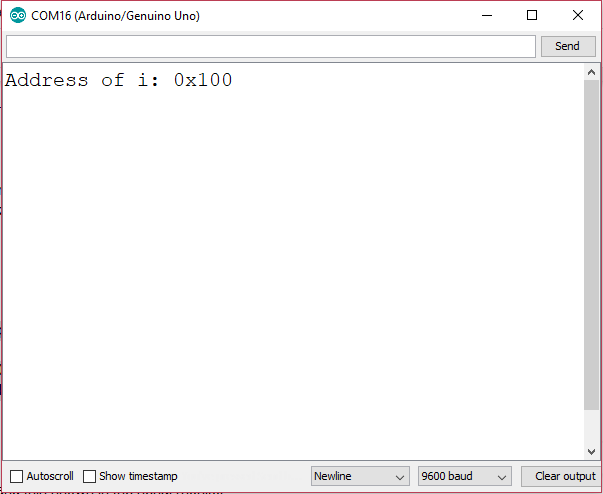This shows that the variable i, an integer type that uses 2-byte space, is stored in the memory location 0x100. Conveniently, 0x100 is the start address of the Arduino's SRAM.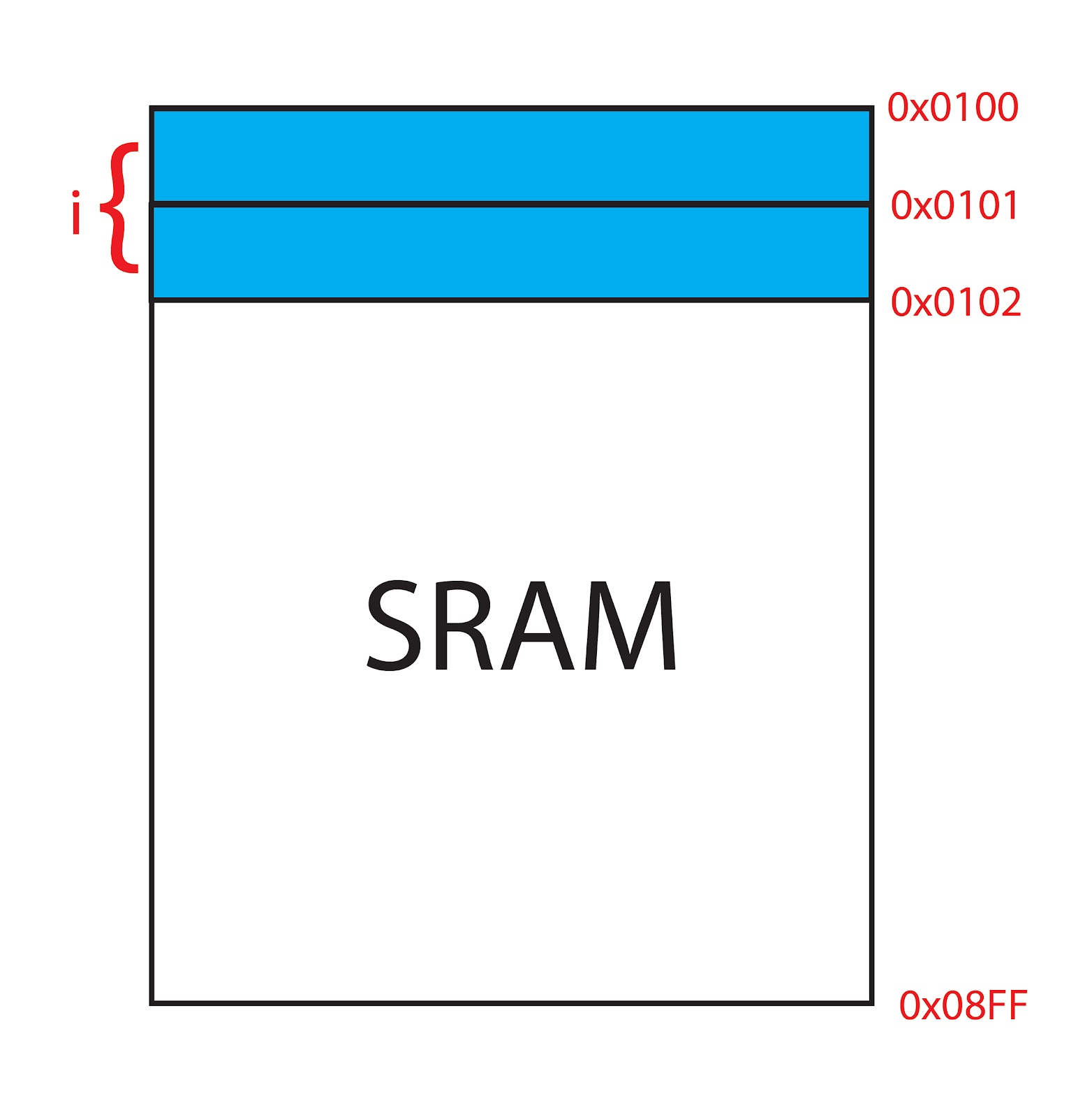This location is reserved for the i variable as it's declared as a global variable being it outside setup() and loop().

Also, note that the pointer variable p itself is using a space in the SRAM. To locate the address of p, we can assign another pointer that points at p:

`int **p = &p;`

The full sketch would be:

```int *p;
int **t = &p;
void setup() {
Serial.begin(9600);
Serial.println((uint16_t)t,HEX);
}

void loop() { }

```

This will print a memory address that is far from that of the address of i. This is because address of pointers are stored in stack.

### Using More than One Pointer

If we are to add another variable and another pointer:

```int *p1;
int *p2;
int i;
int j;
void setup() {
p1 = &i;
p2 = &j;
Serial.begin(9600);
}
void loop() { }```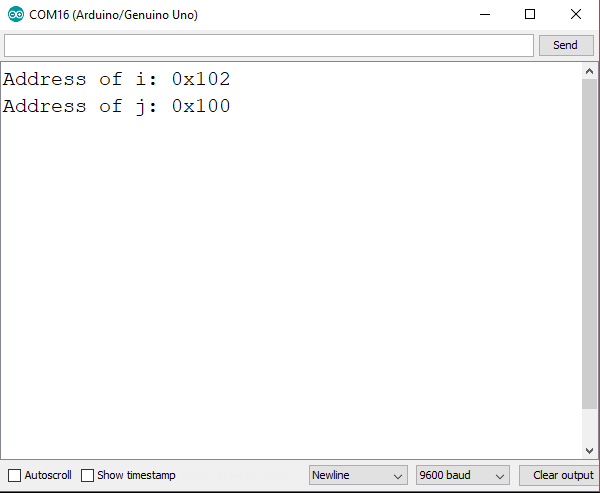Even though that i's declaration came first before j, j takes the 100H address while i takes the 102H address. Both are still global variables and thus take the static data portion of SRAM.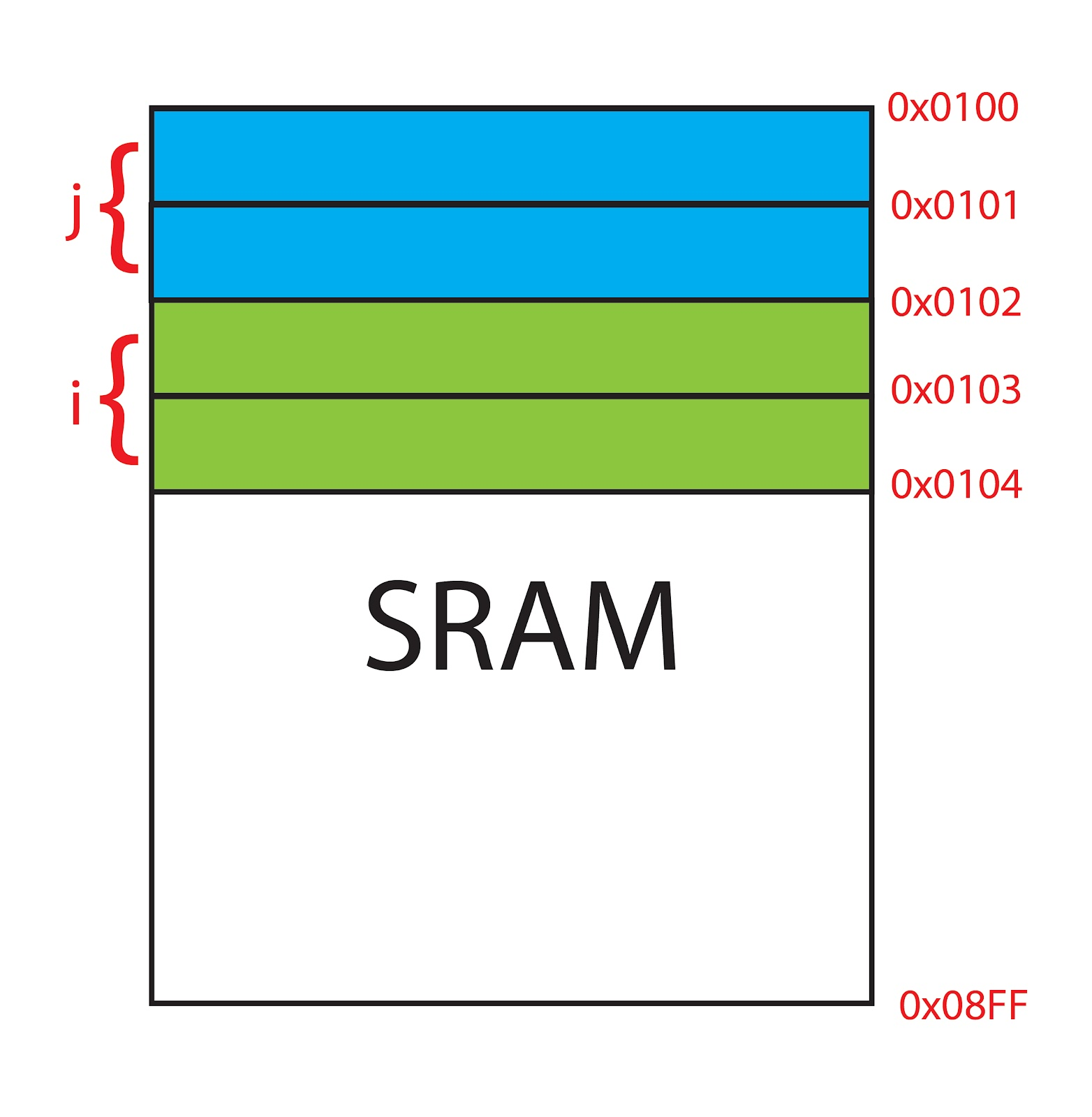### A Pointer to Array

Let’s say we have an array of integers:

`int arr;`

Can we assign a pointer for this array?

If we do it like this:

```int i;
int *p;

void setup() {
p = &i;
Serial.begin(9600);
Serial.println((uint16_t)p, HEX);
}
void loop() {}```

We will have a compiler error:

`cannot convert 'int (*)' to 'int*' in assignment`

This is because i is already the pointer to the array i. To make the above sketch works, we remove the & reference:

```int i;
int *p;

void setup() {
p = i;
Serial.begin(9600);